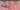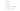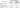# Abnormal Molecular Mass and Vant’s Hoff Factor | Class 12 Chemistry

Posted on October 3rd, 2018

## Abnormal Molecular Mass and Vant’s Hoff Factor | Class 12 Chemistry

Online Class 12th Chemistry Notes Abnormal Molecular Mass and Vant’s Hoff Factor – A substance is said to have an abnormal molecular/molar mass*when the molecular mass of a substance that is determined by measuring any of the colligative properties is different from the theoretically expected value.

*Molecular mass is the sum of the masses of all the atoms present in a molecule. Generally, then it is in the range of 10ˉ²⁷ to 10ˉ²⁵ kg. Molar mass is the mass of one mole of any substance and it is expressed in grams per mole.

We have earlier learned to determine the molecular masses of the solute with the help of colligative properties i.e.relative lowering in vapor pressure, boiling point elevation, freezing point depression, and osmotic pressure. The relation between the molecular mass of solute and colligative properties are determined by considering some assumptions like:

• The solution is diluted to an extent that concentration of solvent is negligible and the solution obeys Raoult’s
• The solute neither undergoes dissociation or association in the solution.

The abnormality in the molecular mass of the solute particles occurs due to either of the following:

• Association of solute particles or
• Dissociation of solute particles

Association of Solute Particles

It is possible that some solute molecules start to associate inside the solution. This suggests, that there are a decreased number of solute particles in the solution. As colligative properties depend on the number of solute particles in the solution, so there will be a decrease in the colligative property along with the solute particles. The colligative properties are inversely proportional to the molecular mass of solute; thus, we get a higher molar mass of the solute. For Example, ethanoic acid or acetic acid (CH3COOH) associate in solution to form a dimer due to hydrogen bonding.

Dissociation of Solute Particles

It is very likely that some solute molecules, generally electrolytes dissociate into two or more ions/particles when they are dissolved in a solution. This causes an increase in solute particles in the solution, hence there is an increase in colligative properties of solutions. As colligative properties and molecular mass of solute vary inversely, hence the molar mass of the solute is decreased.Vant’s Hoff Factor

The Vant’s Hoff factor (named after the Dutch chemist Jacobus Henricus van ‘t Hoff) given by the letter “i” is a measure of the effect of a solute upon colligative properties.

The vant’s Hoff factor is defined as the ratio between the actual concentration of particles (solute) produced when the substance is dissolved in a solvent and the concentration of a substance as calculated from its mass. The van ‘t Hoff factor is basically 1, for most non-electrolytes dissolved in water,

Vant’s Hoff’s factor (i) is also defined as the ratio of the observed colligative property to the calculated colligative property.

i = Observed colligative property / Calculated colligative property

Observed colligative property ∝ 1/Molar Mass

i = Mc/Mo

Vant’s Hoff’s factor (i) tells the extent to which association (or) dissociation of solute molecules take place.

Mathematically, is represented in three forms:

i= Normal molar mass / Abnormal molar mass

= Observed colligative property / Calculated colligative property

= Total number of moles of particles after the association/dissociation /Number of moles of particles before the association/dissociation

Value of i :

For association, i < 1

For dissociation, i > 1

No association or dissociation, i = 1

Degree of dissociation (α): It is the fraction of total molecules which dissociate into simpler molecules or ions. It is given by the symbol α.

α= i – 1 / m – 1

m= number of particles in solution

Degree of association (α): It is defined as the fraction of the total number of solute molecules which combine together to form a bigger molecule.

α=i – 1 / 1/m – 1

m = number of particles in solution.

The table below shows that the different colligative properties change with the dissociation and association of the solute particles in the solvent. The values of the colligative properties are given in terms of vont’s Hoff factor i.For the latest updates regarding government vacancies, results, inquiries, articles, online classes for Class 12, free online test series, register with Takshila Learning.

Follow us on a Social media